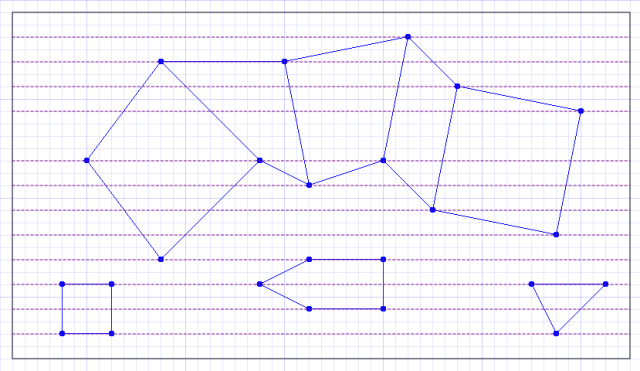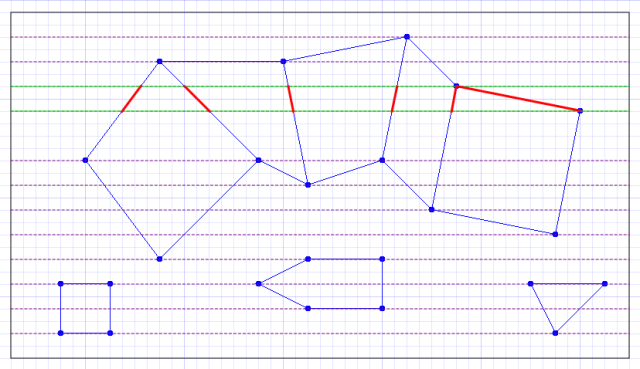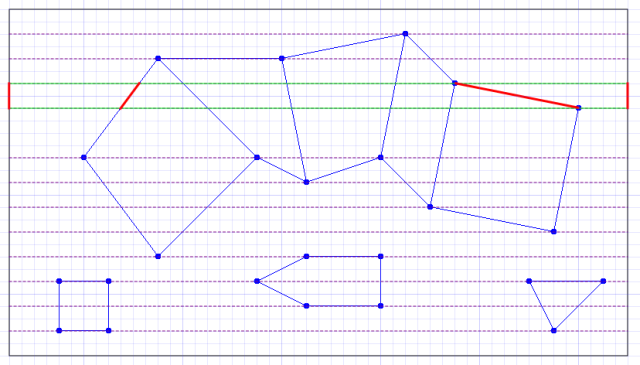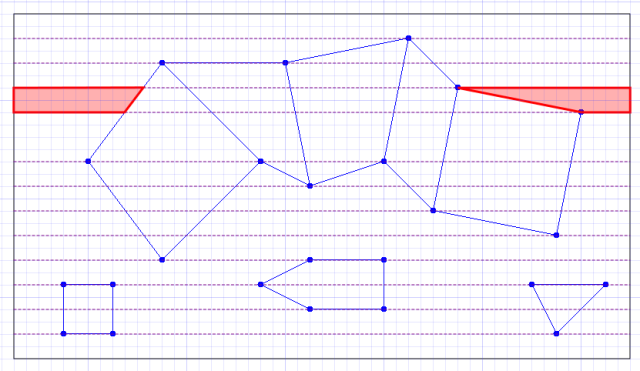# crush depth

Stack Overflow

I actually asked a while back on Stack Overflow if anyone had any idea how to solve the problem I've been attempting to solve with the room model.

Given that I've now actually solved it, I went back to append the answer to my question. I immediately ran into an endless series of idiotically draconian checks that essentially wouldn't let me post the answer to my own question. I eventually gave up trying to get the post to pass the full body cavity search and blood sample analysis, so ended up wrapping the entire thing in `preformatted text` tags and preceding it with a plea that a passing editor fix it. Consider me discouraged from ever bothering to post on Stack Overflow again.

For future reference, here's the solution I posted:

I came up with a solution to this. In order to solve this efficiently, some sort of spatial data structure is needed in order to query which polygons are overlapped by a given rectangular area. I used a Quadtree. It's also necessary for the polygon data structure being used to be able to distinguish between internal and external edges. An edge is internal if it is common to two polygons.

The steps are as follows (assuming a coordinate system with the origin in the top-left corner):

1. Insert all polygons into whatever spatial data structure you're using.

2. Iterate over all polygons and build a list of all of the Y values upon which vertices occur. This has the effect of conceptually dividing up the scene into horizontal strips:3. Iterate over the pairs of Y values from top to bottom. For each pair `(y0, y1)` of Y values, declare a rectangular area `a` with the the top left corner `(0, y0)` and bottom right corner `(width, y1)`. Determine the set of polygons `S` that are overlapped by `a` by querying the spatial data structure. For each polygon `p` in `S`, determine the set of edges `E` of `p` that are overlapped by `a`. For best results, ignore any edge in `E` with a normal that points directly up or down. For each edge `e` in `E`, it's then necessary to determine the pair of points at which `e` intersects the top and bottom edges of `a`. This is achieved with a simple line intersection test, treating the top and bottom edges of `a` as simple horizontal line segments. Join the intersection points to create a set of new line segments, shown in red:4. Create vertical line segments `L0 = (0, y0) → (0, y1)` and `L1 = (width, y0) → (width, y1)`. Working from left to right, gather any line segments created in the preceding step into pairs, ignoring any line segments that were created from internal edges. If there were no intersecting external edges, then the only two edges will be `L0` and `L1`. In this example strip, only four edges remain:5. Join the vertices in the remaining pairs of edges to create polygons:Repeating the above process for each horizontal strip achieves the desired result. Assuming a set of convex, non-overlapping polygons as input, the created polygons are guaranteed to be either triangles or quadrilaterals. If a horizontal strip contains no edges, the algorithm will create a single rectangle. If no polygons exist in the scene, the algorithm will create a single rectangle covering the whole scene.